Get instant live expert help with Excel or Google Sheets“My Excelchat expert helped me in less than 20 minutes, saving me what would have been 5 hours of work!”

#### Post your problem and you’ll get Expert help in seconds.

Your message must be at least 40 characters
Our professional Expert are available now. Your privacy is guaranteed.

# Excel Data Validation Using the YEAR FunctionRead time: 25 minutes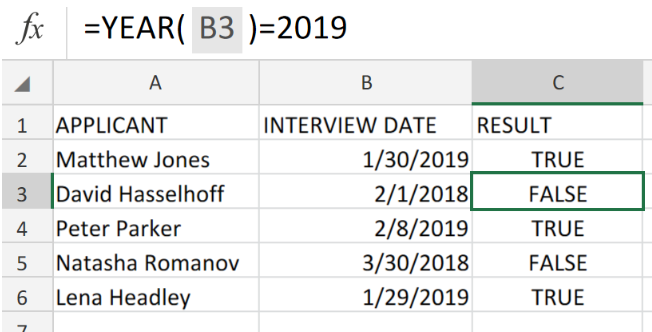Figure 1. Excel Data Validation Using the YEAR Function.

Let’s assume that we have to generate a DATA VALIDATION using Excel YEAR Function to permit entries of dates occurring within a specific year, we must utilize a custom formula syntax based on the YEAR Function in Excel.

## Formula Syntax

`=YEAR(date)`

This formula syntax returns as a result TRUE or FALSE. Which simply means that formula will return FALSE if all conditions in the formula are FALSE, and TRUE when all conditions in the formula are TRUE.

## How to use Excel DATA VALIDATION Using the YEAR Function.

Let’s say we have to create a data entry validation for dates occurring before the year 2019 in Excel,we are going to get this done by following 3 simple steps;

• In our worksheet example below, we have a collection of dates scheduled for interviews;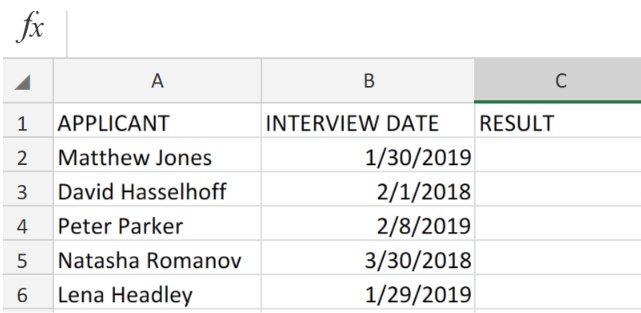Figure 2. Excel Data Validation Using the YEAR Function.

Our purpose here is to flag any date entries that occur before the year 2019.

• The DATA VALIDATION formula that we will enter into cell C2 of our worksheet example is as follows;
`=YEAR(B2)=2019`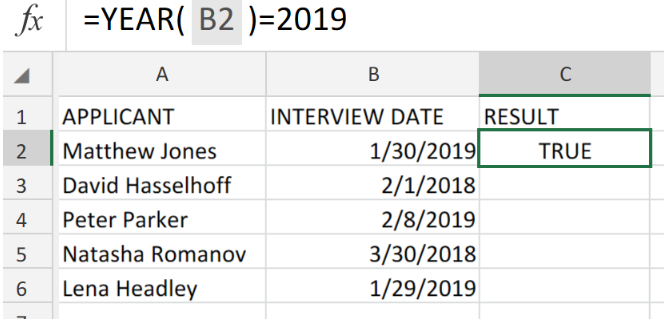Figure 3. Excel Data Validation Using the YEAR Function.

Excel returned the result of the Data Validation as TRUE because the date falls within the year 2019 (assume that today is 27th of January 2019).

• Copy and paste the modified version of the Data Validation formula in cell C2 above, down into the other cells in the RESULT column for the next results.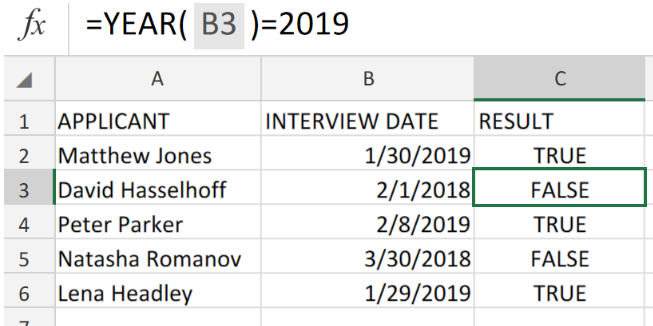Figure 4. Excel Data Validation Using the YEAR Function.

We can utilize the custom data validation formula based on the YEAR function to permit a user to enter only dates in a specific year.

Anytime a user adds or changes a cell value, data validation rules are triggered.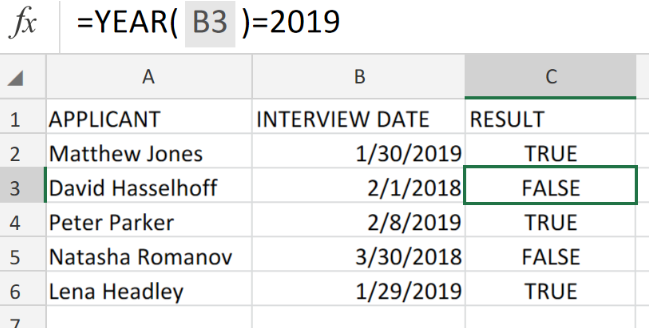Figure 5. Final Result.

## Instant Connection to an Expert through our Excelchat Service:

Our live Excelchat Service is here for you. We have Excel Experts available 24/7 to answer any Excel questions you may have. Guaranteed connection within 30 seconds and a customized solution for you within 20 minutes.

### Did this post not answer your question? Get a solution from connecting with the expert.Another blog reader asked this question today on Excelchat:
Related blogs
Solution examplesUse the Vlookup Function to complete the "employee" column of table 2. Use "job Id" from table 2 as your lookup_value(s) and table 1 as your reference.
Solved by C. H. in 16 minsIf a cell in another sheet is populated I need a vlookup done. If the cell is not populated I need the cell to return blank.
Solved by T. D. in 60 minsI am trying to make a chart that turns a week range red if nothing is entered in the range. If something is entered then I would like it to turn green. Please Help
Solved by E. U. in 43 minsI need a check box to show/hide an answer of an if function
Solved by Z. U. in 23 minsI need a formula to compare the data in two columns and then export the mismatched data in the 3rd column
Solved by S. Q. in 20 mins## Subscribe to Excelchat.coAnother blog reader asked this question today on Excelchat: